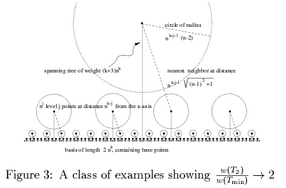## neal young / Fekete97Network

• Journal of Algorithms 24(2):310-324(1997)The problem considered is the following. Given a graph with edge weights satisfying the triangle inequality, and a degree bound for each vertex, compute a low-weight spanning tree such that the degree of each vertex is at most its specified bound. The problem is NP-hard (it generalizes Traveling Salesman (TSP)). The paper describes a network-flow heuristic for modifying a given tree T to meet the constraints. Choosing T to be a minimum spanning tree (MST) yields approximation algorithms with performance guarantee less than 2 for the problem on geometric graphs with Lp-norms. The paper also describes a Euclidean graph whose minimum TSP costs twice the MST, disproving a conjecture made in [Low-degree spanning trees of small weight].